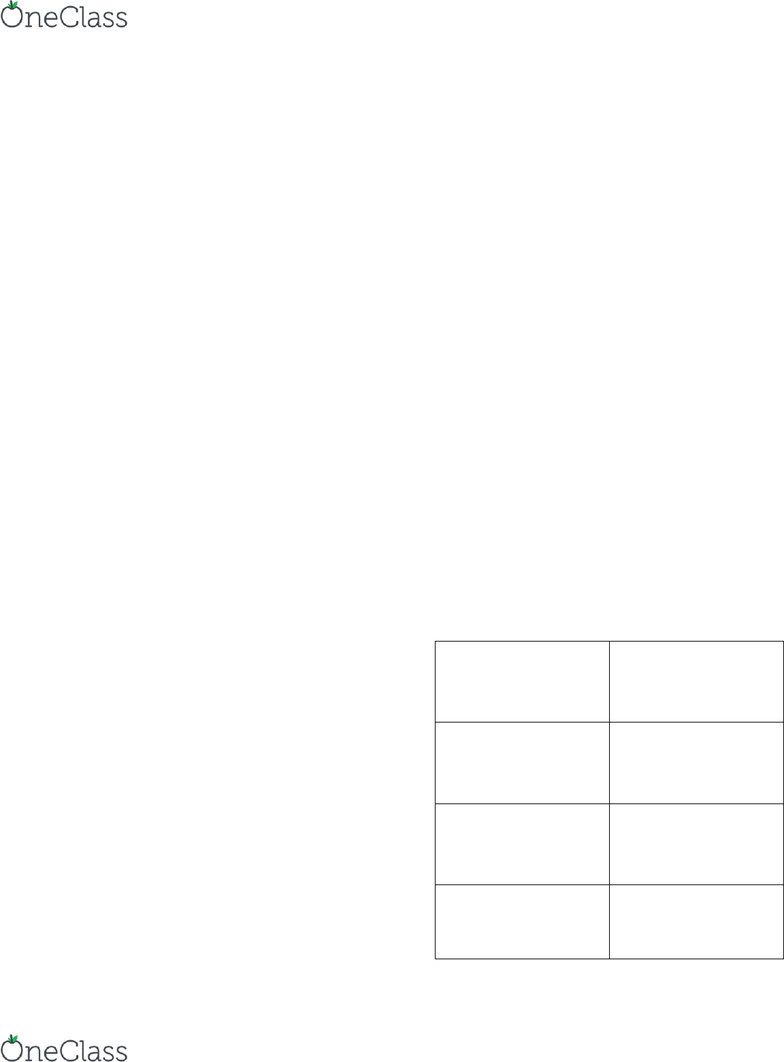Textbook Notes (280,000)
US (110,000)
CHEM (3)
hallen (3)
Chapter 7, 9

# CHEM 101 Chapter Notes - Chapter 7, 9: Zo2, Exothermic Process, Electrolyte

Page:
of 2Exam 3 Study Guide
Chem 101 Hallen
Combination: A + B AB
Decompostition: AB A + B
Single Replacement: A + BC AC + B
Double Replacement: AB + BC AD + CB
Combustion: CxHy + ZO2 XCO2 + y/2 H2O + energy
Loss of Electrons Oxidation (addition of oxygen, loss of hydrogen)
Gaining of Electrons Reduction (loss of oxygen, gain of hydrogen)
LEO goes GER
1 mol = 6.02 x 1023 atoms/molecules
1 mol = g of substance
Percent yield = (actual yield/ theoretical yield) x 100%
Exothermic heat released heat is a product -
Endothermic heat absorbed heat is a reactant +
like dissolves like
Water- polar
Oil- nonpolar
Ionic dissolves in water
Solutions Will Form
Solutions Not Form
Solute Solvent
Solute Solvent
Polar Polar
Polar Nonpolar
Nonpolar Nonpolar
Nonpolar Polar
find more resources at oneclass.com
find more resources at oneclass.com
Eg = +/- charge (1 mol Xn+/-) / (n Eg Xn+/-)
1 Eg = 1000 mEg
Concentration of a solution= amount of solute/amount of solution
Mass Percent (m/m) = mass of solutes/ (mass of solute+ solvent mass)
Volume Percent (v/v) = (volume of solute/volume of solution) x 100%
Mass/ Volume (m/v) = (grams of solute/milliliters of solution) x 100%
Molarity= moles of solute/liters of solution
# of % x/x conversion
% contains # x/x •  conversion
C1V1 (concentrated solution) = C2V2 (diluted solution)
C Molarity or Concentration
Types of
Solute
In Solution
Particles in
Solution
Conducts?
Examples
Strong Electrolyte
Dissociates
completely
Ions only
Yes
Ionic, bases
Weak Electrolyte
Ionizes Partially
Mostly molecules,
few ions
weakly
HF, H2O, NH3, O2
Non Electrolyte
No ionization
Molecules only
No
Carbon Compounds
find more resources at oneclass.com
find more resources at oneclass.com